## Example Questions

### Example Question #150 : Integers

Evaluate:

3 + 2(1 * 9 + 8) – 9/3

34

37/3

36

76/3

82

34

Explanation:

Order of operations

Do everything inside the parenthesis first:

3 + 2(17) – 9/3

next, do multiplication/division

3 + 34 – 3

= 34

### Example Question #11 : Operations

3 + 4 * 5 / 10 – 2 =

5

5.5

3

1.5

7

3

Explanation:

Here we must use order of operations. First we multiply 4 * 5 = 20. Then 20 / 10 = 2. Now we can do the addition and substraction. 3 + 2 – 2 = 3.  If you started at the beginning on the left hand side and not used order of operations, you would mistakenly choose 1.5 as the correct answer.

### Example Question #1 : How To Find Order Of Operations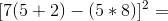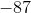Explanation:

Order of operations can be remembered by PEMDAS (Please Excuse My Dear Aunt Sally): Parentheses Exponents Multiplication Division Addition Subtraction.

[7(5 + 2) – (5 * 8)]2 =

1: Inner parentheses   = [ 7(7) – 40 ]2

2: Outer brackets        = [ 49 – 40 ]2 = 92

3: Exponents               = 81

### Example Question #153 : Integers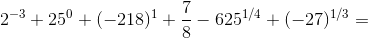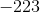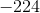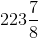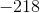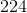Explanation:

This looks daunting as one long equation, but let's look at each piece and then add them all together.

23 = 1/23 = 1/8

250 = 1

(–218)1 = –218

6251/4 = 5

(–27)1/3 = –3

Then, 23 + 250 + (–218)1 + 7/8 – 6251/4 + (–27)1/3 = 1/8 + 1 – 218 + 7/8 – 5 – 3 = –224.

Tired of practice problems?

Try live online GRE prep today.